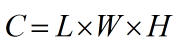Request a Tool

Cubic Feet Calculator

Cubic Feet Calculator is use to calculate cubic feet in ft, in, yd, mm, cm and m units.

Cubic Feet
0

Formula• C = Cubic feet
• L = Value of length
• W = Value of width
• H = Value of height

Defination / Uses

In the United States and the United Kingdom, the cubic foot is an imperial and US customary unit of volume. It's defined as the volume of a cube with one-foot-long sides. It has a volume of 28.3168 litres. A cubic foot of water weighs 62.37 pounds at 60 degrees Fahrenheit.

The volume of a cuboid can be calculated and expressed in cubic feet using a cubic feet calculator. A cuboid is a solid shape with six quadrilateral faces in three dimensions. Enter the values in the input boxes and select the unit from the drop-down list to use the cubic feet calculator. The volume of a space measured in feet is known as cubic footage. It's the volume of a cube with dimensions of one foot in width, length, and height. Cubic feet are mostly utilised in the United States and parts of Canada. For shipping, freight, and landscaping tasks, knowing the cubic footage of an object or location is frequently required.